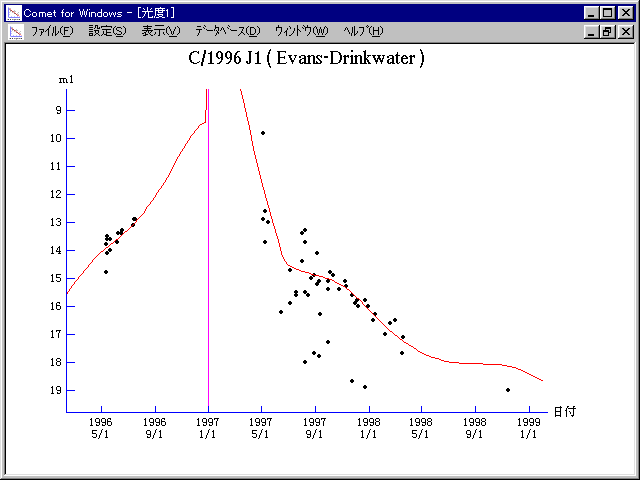# \$B%(%t%!%s%9(B-\$B%I%j%s%/%&%)!<%?!

C/1996 J1 ( Evans-Drinkwater )###\$B%W%m%U%#!<%k(B

 \$BH/8+F|(B 1996\$BG/(B5\$B7n(B10\$BF|(B \$BH/8+8wEY(B 16\$BEy(B \$BH/8+ Robert Evans, M. J. Drinkwater (Siding Spring, Australia)

###\$B50F;MWAG(B

```    Comet C/1996 J1 ( Evans-Drinkwater )  Nucleus - A

The following improved orbital elements, by Kenji Muraoka, are
from 131 observations 1996 May 10 to 1997 May  9, perturbations
by 9 Planets, Moon and 5 minor planets  were taken into account.
The mean residual is +/- 0.76 arc seconds.

Epoch  =  1996 Dec. 23.0  TT       JDT = 2450440.5
T  =  1996 Dec. 30.44848       +/- 0.00316 (m.e.) TT
Peri. =   14.84167                +/- 0.00173
Node  =  278.15627                +/- 0.00173   (2000.0)
Incl. =   22.51948                +/- 0.00038
q  =    1.2978503              +/- 0.0000056 AU
e  =    1.0010150              +/- 0.0000205
1/a  =   -0.0007821              +/- 0.0000158 1/AU
orig. 1/a  =   -0.0000829
fut.  1/a  =   +0.0004871

Comet C/1996 J1 ( Evans-Drinkwater )  Nucleus - B

The following improved orbital elements by Kenji Muraoka, are
from 501 observations 1996 May 10 to 1998 Nov. 14, perturbations
by 9 Planets, Moon and 5 minor planets were taken into account.
The mean residual is +/- 0.68 arc seconds.

Epoch  =  1996 Dec. 23.0  TT       JDT = 2450440.5
T  =  1996 Dec. 30.41829       +/- 0.00055 (m.e.) TT
Peri. =   14.84145                +/- 0.00012
Node  =  278.16709                +/- 0.00011   (2000.0)
Incl. =   22.51861                +/- 0.00004
q  =    1.2975715              +/- 0.0000016 AU
e  =    1.0009079              +/- 0.0000027
1/a  =   -0.0006997              +/- 0.0000021 1/AU
orig. 1/a  =   -0.000001   1/AU
fut.  1/a  =   +0.000569   1/AU
```

###\$B@1?^(B1995\$BG/(B10\$B7n(B10\$BF|!A(B1997\$BG/(B 1\$B7n(B 2\$BF|(B1997\$BG/(B 1\$B7n(B 2\$BF|!A(B1998\$BG/(B 4\$B7n(B27\$BF|(B

###\$B8wEYJQ2=(B

```        m1 =  6.5 + 5 log\$B&\$(B + 10.0 log r  [   ,  0]  (              \$B!A(B1996\$BG/(B12\$B7n(B30\$BF|(B)
m1 = -0.2 + 5 log\$B&\$(B + 27.6 log r  [  0,180]  (1996\$BG/(B12\$B7n(B30\$BF|!A(B1997\$BG/(B 6\$B7n(B28\$BF|(B)
m1 =  9.7 + 5 log\$B&\$(B +  5.0 log r  [180,   ]  (1997\$BG/(B 6\$B7n(B28\$BF|!A(B              )
```##### \$B50F;MWAG\$OB<2,7r<#;a\$N7W;;\$K\$h\$k\$b\$N\$G\$9!#(B \$B@1?^\$O(B StellaNavigator Ver.2.0 for Windows (\$B%"%9%H%m%"!<%D(B \$BJTCx(B / \$B%"%9%-!<=PHG6I4)(B) \$B\$G:n@.\$7\$?\$b\$N\$G\$9!#(B \$B8wEY%0%i%U\$O(BComet for Windows\$B\$G:n@.\$7\$?\$b\$N\$G\$9!#(B